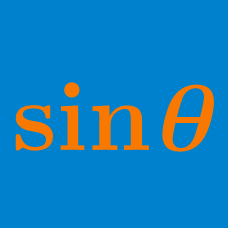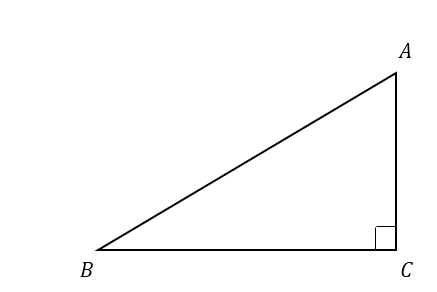Geometry

Basic Trigonometric Functions

In triangle $XYZ$, we have $XY = 14, YZ = 27$ and $ZX= \sqrt{925}$. What is the integer that is closest to $100 \sin \angle XYZ$?

If $\displaystyle \tan A=\frac{3}{4},$ what is the value of $7\cos A ?$If $\angle ACB = 90^\circ,$ $AC=50,$ and $BC= 50\sqrt{3},$ what is $\angle BAC ?$

If $\alpha$ is a real number such that $\tan \alpha = \frac{1}{\sqrt{3}}$ and $\cos \alpha = \frac{\sqrt{3}}{2},$ what is the value of $\frac{1}{\sin \alpha}$?

If $\displaystyle \tan A=\frac{9}{40},$ what is the value of $7\cos A ?$

×